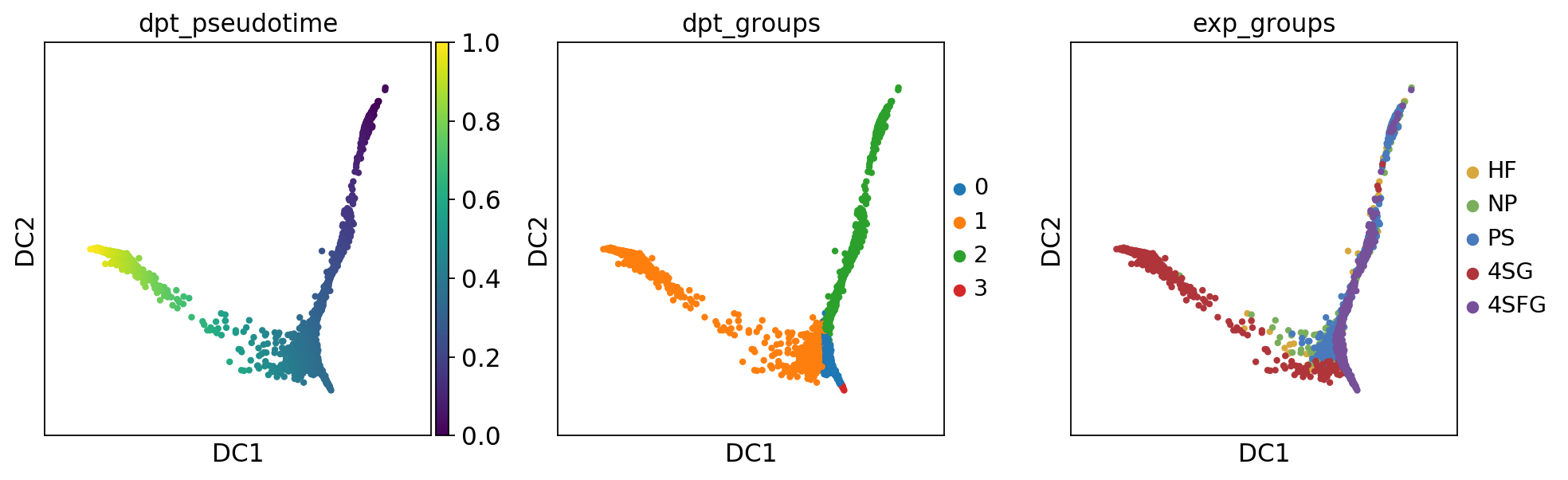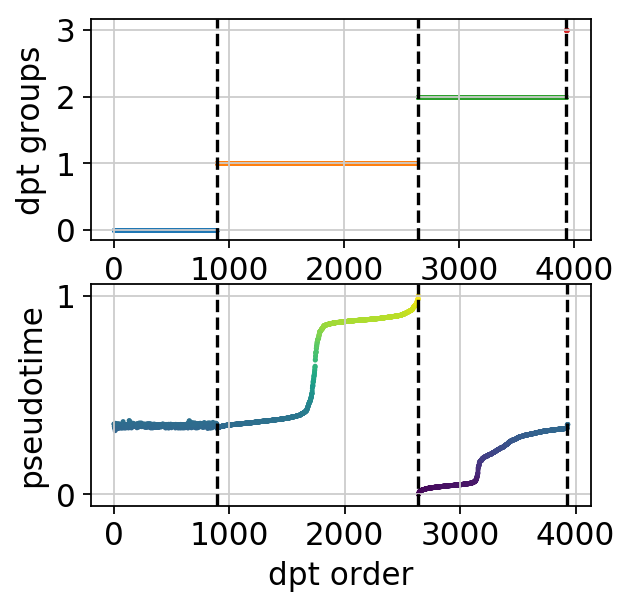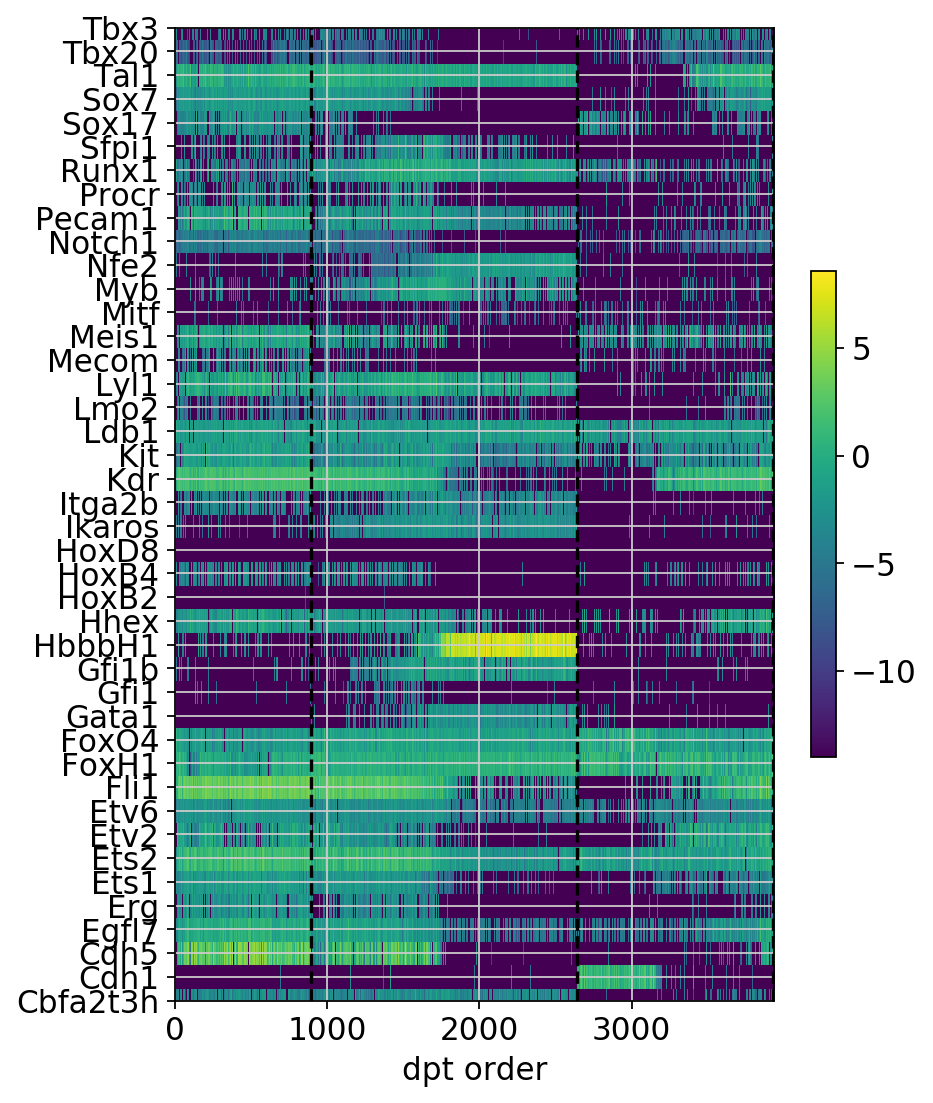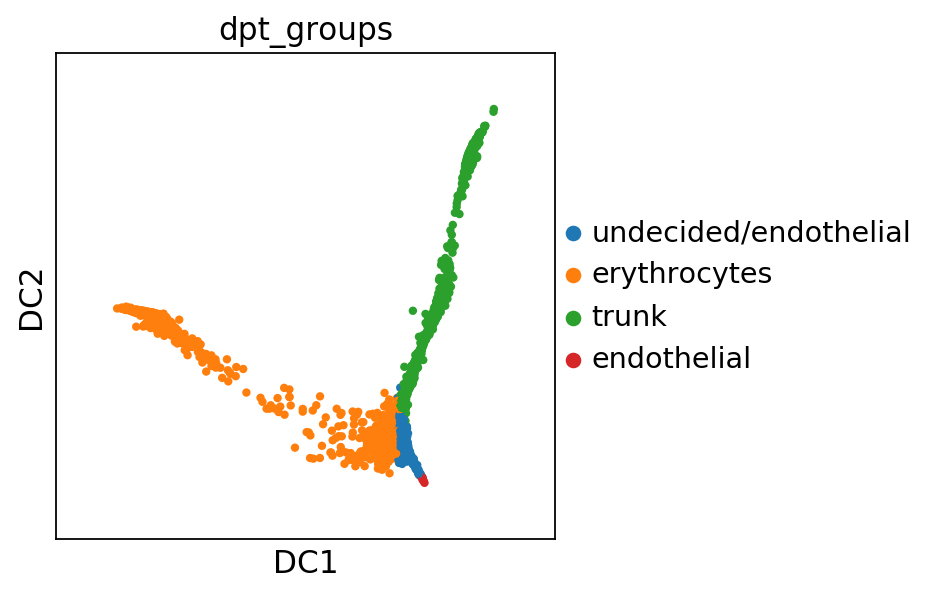# DPT for hematopoiesis in mouse (Moignard et al., 2015)¶

The following analysis has been published by Haghverdi et al., Nat. Meth. (2016) together with a Matlab implementation of Diffusion Pseudotime. The data is from Moignard et al., Nat. Biotechn. (2015).

In :
import numpy as np
import pandas as pd
import scanpy.api as sc

sc.settings.verbosity = 3  # verbosity: errors (0), warnings (1), info (2), hints (3)
sc.logging.print_versions()

scanpy==1.3.1+83.g641af42 anndata==0.6.10+31.g4c40622 numpy==1.14.5 scipy==1.1.0 pandas==0.23.4 scikit-learn==0.19.1 statsmodels==0.9.0 python-igraph==0.7.1 louvain==0.6.1

In :
sc.settings.set_figure_params(dpi=80)  # low dpi (dots per inch) yields small inline figures


This is single-cell qPCR data. It's already on a logrithmic scale and pretty low-dimensional. We do not have to perform extensive preprocessing.

1. Filter out a few genes.
2. Choose a 'root cell'.
3. Define groupnames by inspecting cellnames.
In :
adata = sc.datasets.moignard15()

... writing an h5ad cache file to speedup reading next time


Compute the neighborhood relations of single cells.

In :
sc.pp.neighbors(adata, n_neighbors=5, method='gauss', knn=False)

computing neighbors
using data matrix X directly
finished (0:00:00.98) --> added to .uns['neighbors']


Compute branchings and diffusion pseudotime using DPT.

In :
sc.tl.diffmap(adata)

computing Diffusion Maps using n_comps=15(=n_dcs)
eigenvalues of transition matrix
[1.0000018  0.99249375 0.9739005  0.8865169  0.86071664 0.8255125
0.78051525 0.7599627  0.7338714  0.7300987  0.7136638  0.7079268
0.7056841  0.6916961  0.690119  ]
'diffmap_evals', eigenvalues of transition matrix (adata.uns)

In :
sc.tl.dpt(adata, n_branchings=1, n_dcs=10)

computing Diffusion Pseudotime using n_dcs=10
'dpt_groups', the branching subgroups of dpt (adata.obs)

In :
sc.pl.diffmap(adata, color=['dpt_pseudotime', 'dpt_groups', 'exp_groups'])In :
sc.pl.dpt_groups_pseudotime(adata)In :
sc.pl.dpt_timeseries(adata)Let us annotate the cell groups as follows.

In :
adata.rename_categories('dpt_groups', ['undecided/endothelial', 'erythrocytes', 'trunk', 'endothelial'])

In :
sc.pl.diffmap(adata, color='dpt_groups')Save the results.

In :
adata.write(results_file)


Or as csv.

In :
# adata.obs.to_csv('./write/annotation.csv')

In :
# To write the full object to csvs